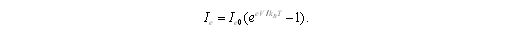# p-n Junction

## Introduction

To understand the majority of semiconductor devices, it is necessary to consider the behaviour of charge carriers near a surface or interface. The key important features are the boundary between an n-type region and a p-type region, the boundary between a semiconductor and an insulator, and the boundary between two different semiconductors.

## p-n JunctionFigure 1: p-n junction in equilibrium. Arrows indicate equal and opposite electron currents Ieo, and hole currents, Iho. This is a diagram of electron energy levels, therefore a region of low energy is a region of high electrostatic potential.

When a thin layer of donor impurities is deposited on the surface of a p-type semiconductor at a controlled period of time with high temperature to allow diffusion of the donors into the substrate, an n-type region is created near the surface where the donors outnumber the original acceptors. Another method of making a p-n junction is by epitaxial growth on a p-type substrate of material containing donor impurities.

In figure 1, the energy levels are shown as a function of position only and no distinction is made between different k-values. Full circles represent electrons in the conduction band and open circles represent holes in the valence band. The equilibrium of a p-n junction is achieved by a small transfer of electrons from the n region to the p region, where they annihilate with holes, leaving region with very few free carriers near the junction, known as the depletion layer.

At the depletion layer, positively charged ionized donors in the n region and negatively charged ionized acceptors in the p region leave the n region positively charged and the p region negatively charged. Hence, a lowering of electron energy levels on the n side and a rise in electron energy levels on the p side. Therefore the chemical potential, µ, becomes position independent.

## p-n Junction with Applied Bias

Electric current flows through a p-n junction by applying a potential difference, V. In forward biased, V is positive and the positive side of the potential is attached to the p region. However, in reverse biased, V is negative and the positive side of the potential is attached to the n region. As in figure 1(b) and (c), forward bias reduces the total potential difference whereas reverse bias increases it. The width of the depletion layer also decreases by forward bias and vice versa.

A small change, dV, in bias can cause the junction to behave as if it has a capacitance per unit area and p-n junctions intended for this use are called varactor diodes or varicaps and can only be used under conditions of reverse bias where the current flow is very small.

The effect of forward bias, as shown in figure 1(b) is to reduce the potential barrier by an amount eV. There is an increase of a factor of exp(eV/kBT) of electrons on the n side with sufficient energy to overcome the barrier since the Boltzmann distribution gives the occupancy of electron states within the conduction band. The flow from p to n remains unchanged since there is no potential barrier for motion in this direction, but the electron current will increase from n to p to Ieoexp(eV/ kBT). Figure 1(b) indicates the imbalance in electron currents and the net electron current through the junction becomesThis equation is also applicable for reverse bias where the increase in the potential barrier by e|V| reduces the flow of electrons from n to p by a factor of exp(eV/kBT).

Edited by Wan Maryam Wan Ahmad Kamil